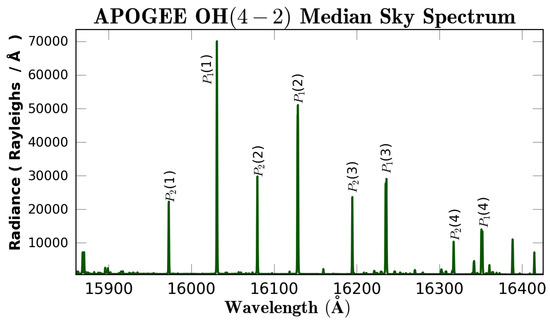Previous Article in Journal
New Gap-Filling Strategies for Long-Period Flux Data Gaps Using a Data-Driven Approach
Open AccessArticle

A Comparison of Einstein A Coefficients for OH Rotational Temperature Measurements Using a Large Astronomical Data Set

byMurdock HartDepartment of Astronomy, Boston University, 725 Commonwealth Ave, Boston, MA 02215, USA
Atmosphere 2019, 10(10), 569; https://doi.org/10.3390/atmos10100569
Received: 10 July 2019 / Revised: 19 September 2019 / Accepted: 20 September 2019 / Published: 22 September 2019
The Einstein A coefficients are considered to be a significant source of uncertainty in the measurement of $OH$ rotational temperatures. Using simultaneous ground and spaced-based observations of $OH$ emission, five sets of Einstein A coefficients were examined for their impact upon rotational temperature calculations. The ground-based observations are taken from the Apache Point Observatory Galactic Evolution Experiment (APOGEE) instrument which is a high resolution, $r = λ$ / $Δ λ$ $≥ 20 , 000$ , spectrograph operating in the H-band from approximately 1.5 to 1.7 $μ$ m. APOGEE collected over one-hundred-and-fifty-thousand spectra of the night sky over a period from June 2011 to June 2013. The Sounding of the Atmosphere using Broadband Emission Radiometry (SABER) instrument on board the Thermosphere Ionosphere Mesosphere Energetics and Dynamics (TIMED) satellite has made simultaneous atmospheric measurements with the APOGEE spectrograph. SABER observes the $OH$ volume emission rate (VER) around 1.6 $μ$ m, providing measurements coincident with those of the $OH$ emission in the APOGEE sky spectra. Four of the five sets of Einstein A coefficients tested yielded statistically identical mean rotational temperatures of approximately 195 K for the $OH ( 4 − 2 )$ transition. The Einstein A coefficients were found to have a significant impact upon the measured $OH ( v ′ = 4 )$ vibrational populations with some sets of coefficients yielding populations over 50% greater. Simultaneous SABER observations were used to determine which set of Einstein A coefficients best reflected atmospheric temperatures, and four of the five tested coefficients yielded nearly identical results. The difference between $OH$ rotational temperatures and SABER temperatures was on average 1 K. View Full-Text
Keywords:
Show FiguresFigure 1

MDPI and ACS Style

Hart, M. A Comparison of Einstein A Coefficients for OH Rotational Temperature Measurements Using a Large Astronomical Data Set. Atmosphere 2019, 10, 569.

Show more citation formats Show less citations formats
Note that from the first issue of 2016, MDPI journals use article numbers instead of page numbers. See further details here.

1